FINANCIAL CHRONICLE™

Registration with the Sri Lanka FINANCIAL CHRONICLEwould enable you to enjoy an array of other services such as Member Rankings, User Groups, Own Posts & Profile, Exclusive Research, Live Chat Box etc..

All information contained in this forum is subject to Disclaimer Notice published.

Thank You
FINANCIAL CHRONICLEwww.srilankachronicle.com

Join the forum, it's quick and easy

FINANCIAL CHRONICLE™

Registration with the Sri Lanka FINANCIAL CHRONICLEwould enable you to enjoy an array of other services such as Member Rankings, User Groups, Own Posts & Profile, Exclusive Research, Live Chat Box etc..

All information contained in this forum is subject to Disclaimer Notice published.

Thank You
FINANCIAL CHRONICLEwww.srilankachronicle.com
FINANCIAL CHRONICLE™
Would you like to react to this message? Create an account in a few clicks or log in to continue.
FINANCIAL CHRONICLE™

Encyclopedia of Latest news, reviews, discussions and analysis of stock market and investment opportunities in Sri Lanka

LISTED COMPANIES
CONTRIBUTE
Poll

#### EXCHANGE RATE PREDICTION: 2022

View results

ශ්‍රී ලංකා මූල්‍ය වංශකථාව - සිංහල
CONATCT USCHRONICLE™ANALYTICS

### Latest topics

» Misuse of government property by Royal Colombo Golf Club
by ChooBoy Yesterday at 12:52 pm

» SL Cauvery and Mannar Basins Petroleum Resource Data Revealed
by samaritan Yesterday at 12:00 pm

» Expolanka to continue its winning streak
by samaritan Yesterday at 10:39 am

» Container shipping stocks routed
by target1 Fri Jun 24, 2022 1:32 pm

» Supply Chain Latest: Ocean Freight Rates Are Falling
by target1 Fri Jun 24, 2022 1:26 pm

» Turkish ship leaves Ukraine's Mariupol after grain talks with Moscow
by target1 Fri Jun 24, 2022 11:29 am

» This week's CBOT corn, soy freefalls hard to match in recent years
by target1 Fri Jun 24, 2022 9:57 am

» Positive news to boost market sentiment
by samaritan Fri Jun 24, 2022 9:52 am

» Maharaja at IMF meeting
by Maharaja Thu Jun 23, 2022 10:35 pm

» Why no power cuts in certain areas of Colombo?
by samaritan Thu Jun 23, 2022 5:44 pm

» India assures fullest support to Sri Lanka as a close friend
by samaritan Thu Jun 23, 2022 5:17 pm

» SINS, SAMP, COMB, PARQ, ALUMEX and AEL - are they good shares to start collecting now?
by surfjj Thu Jun 23, 2022 4:25 pm

» Learn how a fixed exchange rate system can be used to reduce inflation.
by target1 Thu Jun 23, 2022 3:35 pm

» Debt Crisis: Sri Lanka Vs Argentine
by CHRONICLE™ Thu Jun 23, 2022 8:17 am

» Sri Lanka in state of panic 1 month after sovereign default
by reyaz Tue Jun 21, 2022 5:23 pm

» Financial stability - we are at the crossroads.
by sheildskye Tue Jun 21, 2022 2:17 pm

» Shrinkflation: Is it ethical?
by sheildskye Tue Jun 21, 2022 2:05 pm

» Banking bullish billionaire Ishara expands footprint
by samaritan Tue Jun 21, 2022 10:58 am

» SL hopes to finalize a staff level program with IMF soon-RW
by samaritan Tue Jun 21, 2022 9:48 am

» Sri Lanka Stock Market heading toward ASPI 4500
by samaritan Tue Jun 21, 2022 9:29 am

EXPERT CHRONICLE™

RECOMMENDATIONS
MARKET CHAT

CHRONICLE™ ANALYTICS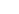MARKET SENTIMENTS & PREDICTIONS
ECONOMIC CHRONICLE

LATEST TWEETS

# Calculating Stock Prices

+2
amilaela
sriranga
6 posters

#### srirangaDear Members,

I read the following article on the internet, which i wish to share with you.
May be it will help us.

Calculating Today's Stock Prices

Since investors buy stocks for both the dividends they pay today, as well as the possibility of a gain in a stock's selling price, then the expected return can be expressed by the following calculation:
Expected Return = (Dividends Paid + Capital Gain) / Price of Stock
This expected return for a stock is also known as the market capitalization rate or discount rate. We're going to use all three terms interchangeably throughout our calculations and explanations in this article. Let's look at a quick example of how this formula works.
Listed below are the starting assumptions:
• Price of Stock A is currently \$100.00 per share or (P0).
• Dividends are expected to be \$3.00 per share (Div).
• The price of Stock A is expected to be \$105.00 per share in one year's time (P1). Therefore our capital gain is expected to be \$105.00 - \$100.00 or \$5.00 per share.
In this example:
Expected Return, or R = (\$3.00 + \$5.00) / \$100.00 = 8.0%
We can now use this expected return to calculate the price of a stock in the same risk class as Stock A using the following formula:
Stock Price = (Dividends Paid (Div) + Expected Price (P1)) / (1 + Expected Return (R))
Proving this calculation with our example information above, we have:
Stock Price = (\$3.00 + \$105) / (1 + 0.08) = \$108.00 / 1.08 = \$100
Some of you may recognize this stock price calculation as the beginnings of a discounted cash flow formula. Essentially, the price of a stock is the cash flows gained by the stockholder, divided by the discount rate or market capitalization rate.

Discounted Cash Flows and Stock Pricing
The stock prices we calculated are really just short term values - a one year horizon. But let's think about the value of a stock over a nearly infinite timeline. Let's say a stockholder plans to sell their stock in 100 years. In this example, the value of the stock would be all of the dividends received each year, plus the capital gain of the stock in 100 years.
We know from our prior example that the investor's expected return was 8.0%, and the growth rate of the stock was 5.0%. In 100 years, the stock's price would then be:
\$100 x (1.05)^100 = \$13,150.13
If we discount this selling price by the expected return, or discount rate, we find:
\$13,150.13 / (1.08)^100 = \$13,150.13 / 2,119.76 = \$5.98
The above information tells us that the current price of a stock has very little to do with the future price of the stock. The net present value of the stock's price 100 years from now is only \$5.98! We could run through all the calculations, but \$94.02 (\$100.00 - \$5.98) of the stock's price is derived from the present value of the dividend received each year.
In fact, when taken to the extreme (an infinite timeline), the price of stock today has no relationship to a future capital gain. It is a function of the dividend stream, divided by the rate of return that can be derived from stocks of similar risk. This allows us to simplify our stock pricing formula:
Stock Price = Sum of Dividends (Div) in each Time (T) / (1 + R)^T
You might recognize this formula as the discounted cash flow formula, where stock dividends are substituted for cash flows.

Stock Pricing via Constant Growth Model
We can further simplify this stock price formula by applying a constant growth model to the company's dividends. This is similar to simplifications that are used in evaluating returns in perpetuity. Using this model, our stock price formula then becomes:
Stock Price = Dividends (Div) / (Expected Return (R) - Dividend Growth Rate (G))
Or
Stock Price = Div / (R - G)
This constant growth stock pricing model does not mean the stock's dividends will remain the same over time; the assumption is that the growth rate is constant over a long period of time. By examining this stock pricing formula, you'll see that it only works when the expected return, or discount rate, is greater than the dividend growth rate. An assumption that is quite logical.
Estimating Market Capitalization and Dividend Growth Rates
Now that we have a simple formula to calculate a stock's price, we need to figure out how to calculate all of the individual variables in that formula. Specifically, we need to calculate the projected growth rate in dividends and the market capitalization rate (discount rate or expected return).

Estimating Dividend Growth Rates
An estimate of a company's dividend growth rate can be made by examining a company's projected earnings growth rate. This estimate assumes that the return on equity for a company and its payout ratio remain constant.
Dividend growth can then be estimated using the following calculation:
Dividend Growth (G) = Plowback Ratio x Return on Equity
Where:
• Plowback Ratio = 1 - Payout Ratio, and
• Payout Ratio = Dividends Paid / Earnings per Share, and
• Return on Equity = Earnings per Share / Book Equity per Share
All of these variables can be easily calculated when you're researching a stock. They are often calculated for you by many of the online stock research tools. We explain the significance of many of these variables in our article on financial ratios.
Sticking with our example, if Stock A has a payout ratio of 60%, which means they pay out 60% of earnings in terms of dividends, their plowback ratio is 1 - 60% or 40%. Let's also assume the company's return on equity is 10.0%. That means their estimated dividend growth rate is:
Dividend Growth (G) = 40% x 10% = 4.0%

Estimating Market Capitalization Rates
If we go back to our simplified stock price formula, we can use that same calculation to develop an estimate of the discount rate (or market capitalization rate). Rearranging this formula we have:
Discount Rate (R) = (Dividends (Div) / Stock Price (P0)) + Dividend Growth Rate (G)
If we are going to develop estimated prices for stocks, then we're going to need to figure out the proper discount rate (expected stockholder return), based on stocks of equivalent risk. That means we need to calculate the discount rate for stocks that are of equivalent risk to the one we're thinking about buying.
We've already discussed how the dividend growth rate can be calculated, so we only need to solve for this portion of the discount rate equation:
Dividends / Stock Price
Fortunately, this particular ratio is a commonly published stock ratio, and is known as the dividend yield. In this example, we are examining a stock of equivalent risk to Stock A. Let's assume that Stock B has:
• Dividend Yield of 7.0%
• Payout Ratio of 45%
• Return on Equity of 12%
First, solving for the dividend growth rate:
Dividend Growth (G) = 55% x 12% = 6.6%
Finally, solving for the discount rate:
Discount Rate (R) = 7.0% + 6.6% = 13.6%
We now have a method for calculating a stock's price based on some fundamental information about the stock itself, and information on stocks of equivalent risk. That is, we've explained how to calculate all of the variables in our stock pricing formula:
Stock Price = Div / (R - G)

Drawbacks of the Constant Growth Stock Pricing Method
The simple discounted cash flow approach to pricing stocks is extremely useful in valuing and evaluating stocks. Whenever estimating stock prices, you should carefully examine the output of any calculation.
For example, the value of the discount rate is very important, and needs to be made for stocks of equivalent risk. The estimate should be based on a reasonably high (ten plus) number of stocks.
Analysts also need to pay careful attention to the growth rates being used. If the company being evaluated has a relatively high growth rate, then the analyst needs to think about the sustainability of the growth rate over time.
Finally, keep in mind that the stock market is a very efficient market. If the stock prices you're calculating are very different than the actual market prices, then you need to take a second look at your assumptions. There is no such thing as easy money when it comes to picking stocks. If there was, we'd all be rich.

http://www.money-zine.com/Investing/Stocks/Calculating-Stock-Prices/

#### amilaelaSenior Manager - Equity Analytics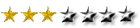thankssss

#### K.HaputantriThanks for sharing Shri.

#### Buzzingstreet

Your research regarding the calculating stock market is good.If you want tips for the stock market then you can definitely contact to our analyst team.

#### jehovahEquity Analytic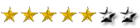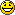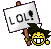#### EquityChampModeratorYou are doing a good job. sriranga's articles are of very valuable.

Thanks mate.

#### jehovahEquity AnalyticEquityChamp wrote:You are doing a good job. sriranga's articles are of very valuable.

Thanks mate.

thank you ec.....Permissions in this forum:
You cannot reply to topics in this forum